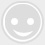# 读懂这篇文章,等于读懂中国股市,让你少走弯路

-回复 -浏览1、打压吸筹建仓

2、推土机建仓法

3、疾风暴雨式建仓法

4、假破底建仓法

5、低位加码型建仓法

6、横盘型建仓法

1.技术表现：量堆

2.技术表现：影线试盘

3.技术表现：缩量下跌

4.技术表现：上升三角形整理

5.技术表现：委比大买单多

6.技术表现：关键价位

7.技术表现：重心不下移式洗盘

8.技术表现：横盘整理

9.技术表现：箱型、旗型整理洗盘

10.技术表现：W底、头肩底震荡洗盘

N:=9;

M1:=3;

M2:=3;

M3:=2;

VAR1:=(HHV(HIGH,N)-LLV(LOW,N));

VAR2:=(HHV(HIGH,N)-CLOSE);

VAR3:=(CLOSE-LLV(LOW,N));

VAR4:=VAR2/VAR1*100-70 ;

VAR5:=(CLOSE-LLV(LOW,60))/(HHV(HIGH,60)-LLV(LOW,60))*100;

VAR6:=(2*C+H+L)/4;

VAR7:=SMA((VAR3/VAR1*100),3,1);

VAR8:=LLV(LOW,34);

VAR9:=SMA(VAR7,3,1)-SMA(VAR4,9,1);

VAR10:= IF(VAR9>100,VAR9-100,0);

VARA:=HHV(HIGH,34);

B1:=EMA(防线,5);

{STICKLINE(防线>0 AND 防线-B1>=0,49,51,2,0),COLORMAGENTA;}

50,LINETHICK2, COLORWHITE;

STICKLINE(防线>0 AND 防线-B1<0,49,51,2,0),COLORYELLOW;

RSV:= (CLOSE-LLV(LOW,N))/(HHV(HIGH,N)-LLV(LOW,N))*100;

FASTK:=SMA(RSV,M1,1);

K:SMA(FASTK,M2,1),LINETHICK2,COLORRED;

D:SMA(K,M3,1),LINETHICK2,COLORGREEN;